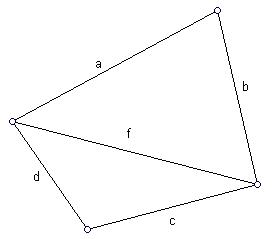Problems

# Heron

Time limit 1 second
Memory limit 128 MiBThe sides a, b, c, d and the diagonal f of a convex quadrilateral are given. Find the area of a quadrilateral, using the Heron’s formula that calculates the area of a triangle.

## Input data

In one line five real numbers are given: a, b, c, d, f (0 < a, b, c, d, f100). They are the lengths of segments as shown in the picture.

## Output data

Print one number - the area of a quadrilateral calculated with accuracy of 4 digits after the decimal point.

## Examples

Input example #1
3 4 4 2 5

Output example #1
9.7997

Source SFE-2010 Variant 26